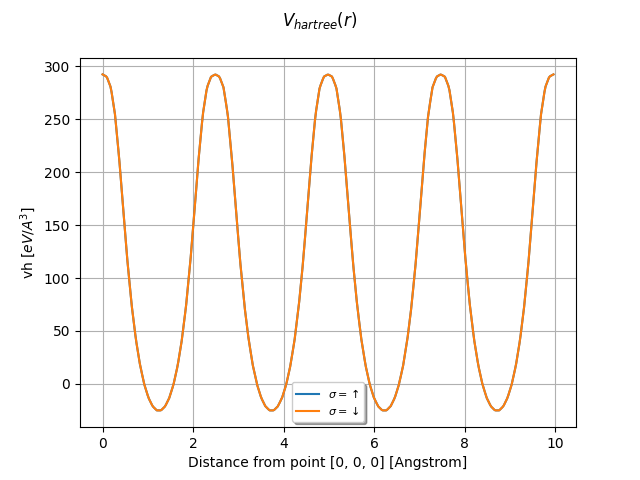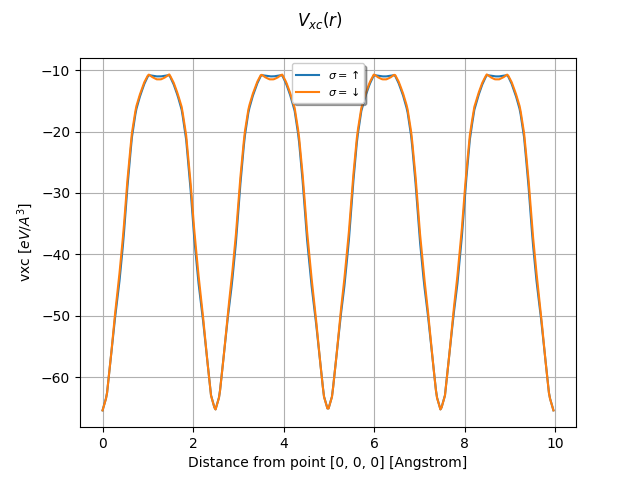# Potentials¶

This example shows how to plot the potentials stored in netcdf files. Use the input variables prtpot, prtvha, prtvhxc, prtvxc with iomode 3 to produce these files at the end of the SCF-GS run.

••••```from abipy.abilab import abiopen
import abipy.data as abidata

# VKS = Hartree + XC potential + sum of local part of pseudos.
with abiopen(abidata.ref_file("ni_666k_POT.nc")) as ncfile:
vks = ncfile.vks

#vks.plot_line(point1=[0, 0, 0], point2=[0, 4, 0], num=400, title="\$V_{ks}(r)\$")

# Hartree potential.
with abiopen(abidata.ref_file("ni_666k_VHA.nc")) as ncfile:
vh = ncfile.vh

vh.plot_line(point1=[0, 0, 0], point2=[0, 4, 0], num=400, title="\$V_{hartree}(r)\$")

# XC potential.
with abiopen(abidata.ref_file("ni_666k_VXC.nc")) as ncfile:
vxc = ncfile.vxc

vxc.plot_line(point1=[0, 0, 0], point2=[0, 4, 0], num=400, title="\$V_{xc}(r)\$")

# Hartree + XC potential.
with abiopen(abidata.ref_file("ni_666k_VHXC.nc")) as ncfile:
vhxc = ncfile.vhxc

vhxc.plot_line(point1=[0, 0, 0], point2=[0, 4, 0], num=400, title="\$V_{hxc}(r)\$")

vloc = vks - vhxc
vloc.plot_line(point1=[0, 0, 0], point2=[0, 4, 0], num=400, title="\$V_{loc}(r)\$")

foo = vhxc - vh - vxc
#foo.plot_line(point1=[0, 0, 0], point2=[0, 4, 0], num=400, title="\$V_{hxc - h - xc}(r)\$")

# To plot the wavefunction along the lines connect the firt atom in the structure
# and all the neighbors within a sphere of radius 3 Angstrom: